# Scientific Notation Scientific Notation way of making REALLY

• Slides: 13Scientific Notation • Scientific Notation – way of making REALLY big or REALLY small numbers easy to read. • General Form: Mx n 10 • M = number between 1 and 10 • n = number of decimal places you move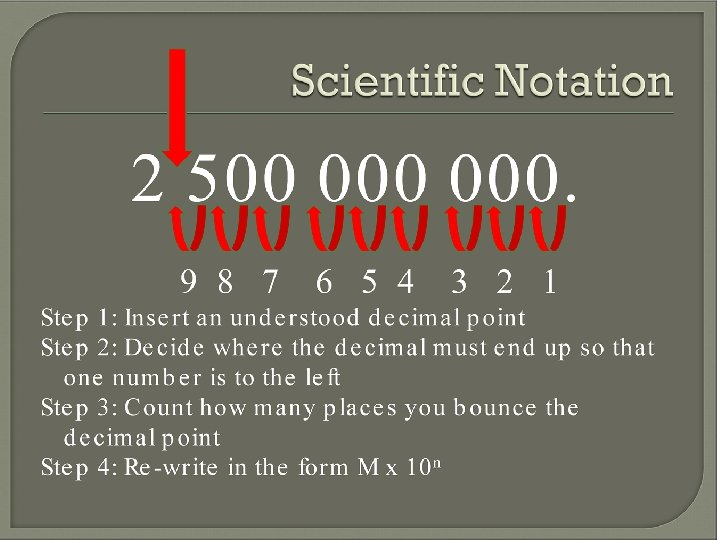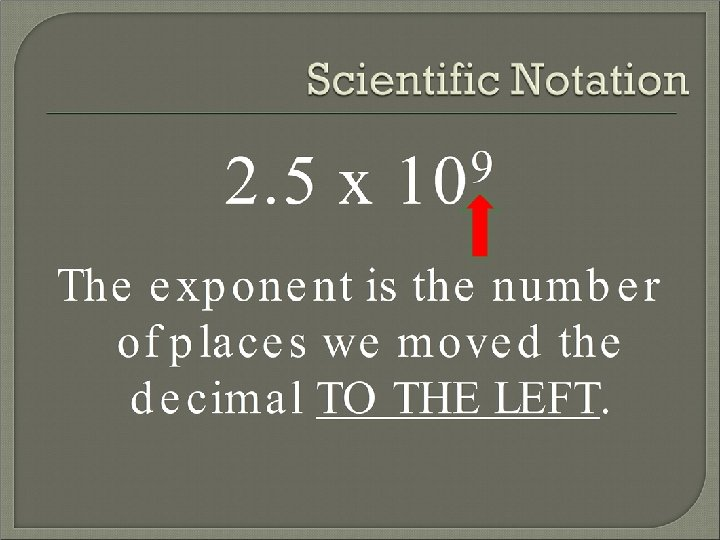Quick Quiz #1 Multiply 123456 x 7890 on your calculator 1. What was your answer (with CORRECT sig figs) 2. Write your answer in scientific notation Do the same for the following problems (with CORRECT SIG FIGS): 1. 98765 x 43210 = ? 2. 10000 x 892800 = ? 3. 90 x 9 = ?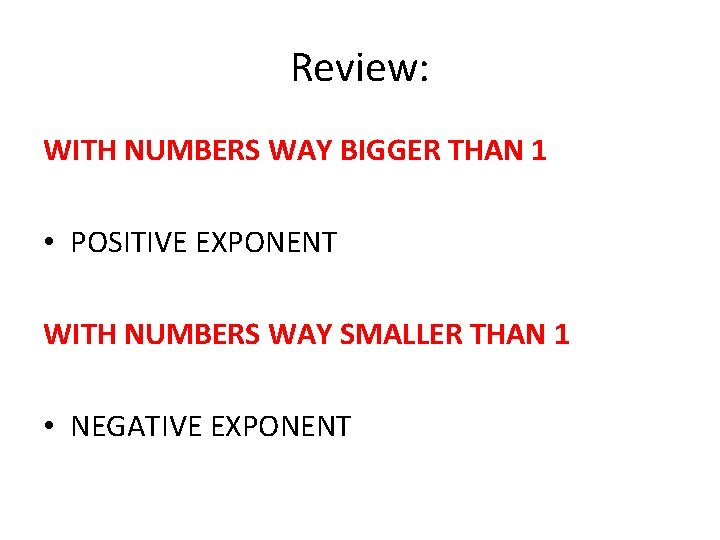Review: WITH NUMBERS WAY BIGGER THAN 1 • POSITIVE EXPONENT WITH NUMBERS WAY SMALLER THAN 1 • NEGATIVE EXPONENT8/31 – Warm Up #3 • I ran a 5 K over the weekend (where K stands for kilometers). Calculate the distance of my run, in centimeters. Additionally convert this answer into scientific notation. • I ran my 5 K in 18 min (the equivalent of 1080 seconds). Calculate the amount of time it takes, in Megaseconds (Msec), and convert your answer into scientific notation.Scientific Notation Reminder: 7. 1 x 104 number of spaces (4 spaces) Size of the number (big number) 7. 1 x 104 71, 000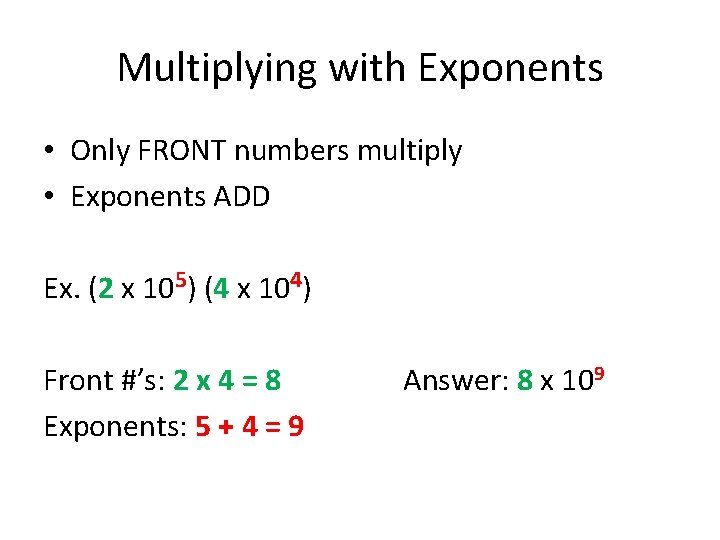Multiplying with Exponents • Only FRONT numbers multiply • Exponents ADD Ex. (2 x 105) (4 x 104) Front #’s: 2 x 4 = 8 Exponents: 5 + 4 = 9 Answer: 8 x 109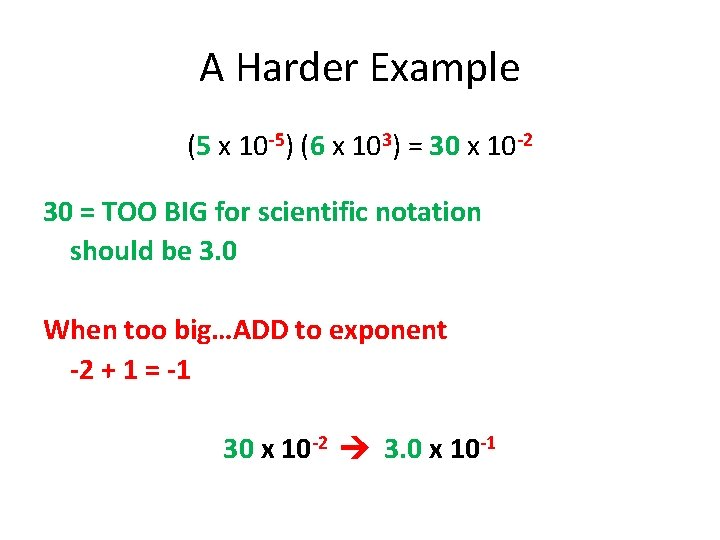A Harder Example (5 x 10 -5) (6 x 103) = 30 x 10 -2 30 = TOO BIG for scientific notation should be 3. 0 When too big…ADD to exponent -2 + 1 = -1 30 x 10 -2 3. 0 x 10 -1Dividing with Exponents • Only FRONT number divide • Exponents SUBTRACT Ex. (6 x 104) (3 x 101) Front #’s: 6/3 = 2 Exponents: 4 – 1 = 3 Answer: 2 x 103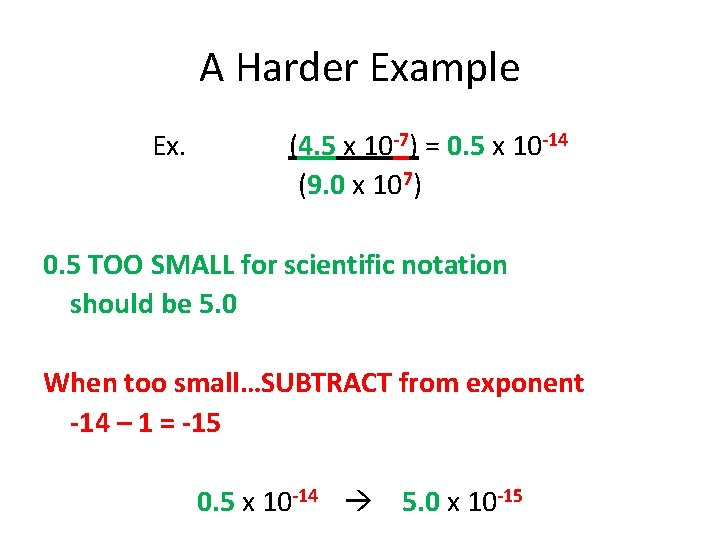A Harder Example Ex. (4. 5 x 10 -7) = 0. 5 x 10 -14 (9. 0 x 107) 0. 5 TOO SMALL for scientific notation should be 5. 0 When too small…SUBTRACT from exponent -14 – 1 = -15 0. 5 x 10 -14 5. 0 x 10 -15